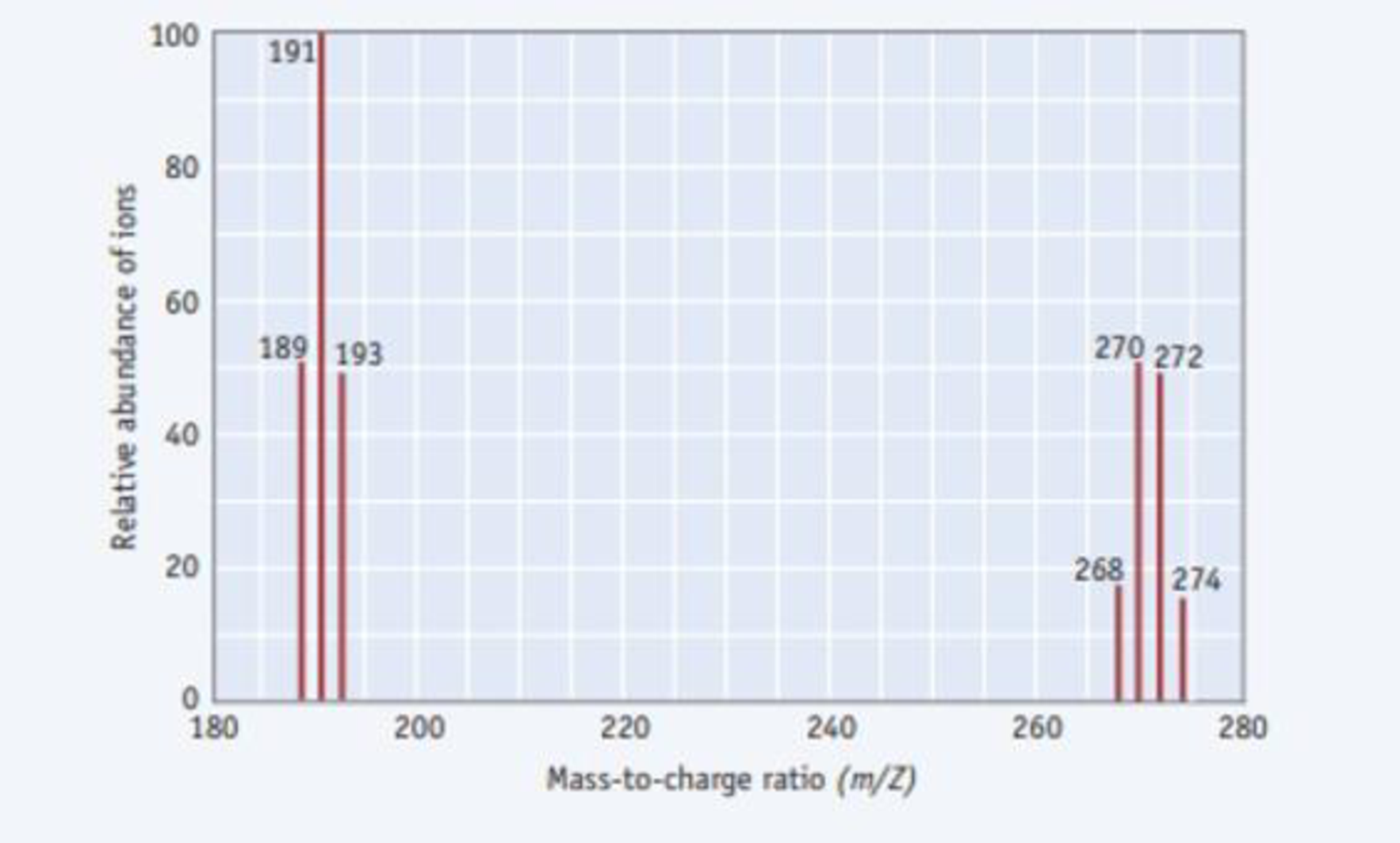Chapter 2.8, Problem 2.12CYU

Chapter
Section
Textbook Problem

The mass spectrum of phosphorus tribromide is illustrated below. Bromine has two stable isotopes, 79Br with abundances of 50.7% and 49.3%, respectively.(a) What molecular species give rise to the parent ion peaks at m/Z ratios of 270 and 272 (b) Explain why the relative abundances of the ions at m/Z ratios of 268 and 274 are approximately one-third of those at 270 and 272.

(a)

Interpretation Introduction

Interpretation:

For the mass spectrum of phosphorus tribromide, the molecular species give peaks at m/Z ratios of 270 and 272 has to be determined

Concept introduction:

Mass spectroscopy: It is a form of spectroscopic technique which is used for the elucidation of the molecules, depending upon the mass of the molecule.

Parent ion peak: It is defined as the prominent peak in the mass spectrum of the molecule which represents the not fragmented molecular ion in the given molecule with greater m/z value.

Base peak: It is the tallest peak in the spectrum.

PBr3 ionize to form positive ions. Some of them fragment into smaller ions.

Elements present in PBr3 are 79Br,81Brand31P

Explanation

m/Z ratios of 270 and 272 shows prominent peaks, so they are PBr3+

The elements present in PBr3 are 79Br,81Brand31P, so the m/Z (charge is taken as 1)

ratios of 31P and two different combination of 79Brand81Br are,

m/Zratio:31Pand2×<

(b)

Interpretation Introduction

Interpretation:

The relative abundance of ions at m/Z ratios of 268 and 274 are approximately one-third of those at 270 and 270 has to be explained.

Concept introduction:

Mass spectroscopy: It is a form of spectroscopic technique which is used for the elucidation of the molecules, depending upon the mass of the molecule.

Parent ion peak: It is defined as the prominent peak in the mass spectrum of the molecule which represents the not fragmented molecular ion in the given molecule with greater m/z value.

Base peak: It is the tallest peak in the spectrum.

PBr3 ionize to form positive ions. Some of them fragment into smaller ions.

Elements present in PBr3 are 79Br,81Brand31P.

Still sussing out bartleby?

Check out a sample textbook solution.

See a sample solution

The Solution to Your Study Problems

Bartleby provides explanations to thousands of textbook problems written by our experts, many with advanced degrees!

Get Started

Find more solutions based on key concepts